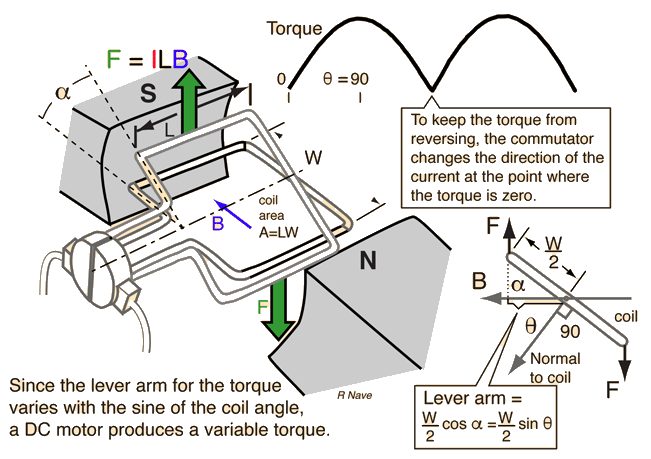# NTNUJAVA: Virtual Physics Laboratory: Enjoy the fun of Physics

You need a java enabled browser (clickfor more information)

• what is the voltage across the split ring commutator when the loop is rotating? by:lookangat 2009/11/03 15:14i am doing some research on java applet simulation on DC motor and AC generator.
http://www.walter-fendt.de/ph11e/generator_e.htm
show an interesting animation of the Dc voltage that resembled a full wave rectification. is that correct?
i thought it will be a constant voltage.

the reason is
i am trying to create a Voltage vs time graph for http://www.phy.ntnu.edu.tw/ntnujava/index.php?topic=1266 and i am puzzled what shape it will be like?

ohm law V =IR,
during closed circuit,
if I is constant, and R is a fixed quantity across the split ring, should V is constant?
during open circuit,
I = 0, imply V = V as it is the voltage of the battery
so is it a constant line of voltage V = battery emf?

or is the physics of electromagnetic induction at the loop more complex that and i forgot to consider?

See 4 replies click1. Re: what is the voltage across the split ring commutator when the loop is rotating? by:hwangat 2009/11/03 21:58I think the magnetic field is assumed to be uniform B=B[sub]0[/sub].
The magnetic flux pass through the coil depends on the angle $\theta=\omega t$,
assume the coil rotate at constant angular velocity $\omega$.
i.e. $\Psi=\int \vec{B}\cdot d\vec{s}= B A cos(\theta)=B A cos(\omega t)$
From Faraday's law, induced voltage $V= -\frac{d\Psi}{dt}= BA\omega \sin(\omega t)$
So the voltage is a sine wave (without commutator).

2. Re: what is the voltage across the split ring commutator when the loop is rotating? by:lookangat 2009/11/03 22:33[quote author=Fu-Kwun Hwang link=topic=1281.msg4947#msg4947 date=1257256703]
I think the magnetic field is assumed to be uniform B=B[sub]0[/sub].
The magnetic flux pass through the coil depends on the angle $\theta=\omega t$,
assume the coil rotate at constant angular velocity $\omega$.
i.e. $\Psi=\int \vec{B}\cdot d\vec{s}= B A cos(\theta)=B A cos(\omega t)$
From Faraday's law, induced voltage $V= -\frac{d\Psi}{dt}= BA\omega \sin(\omega t)$
So the voltage is a sine wave (without commutator).
[/quote]
i agree with the above analysis that AC generator (without commutator) the emf induced in the coil is a sine wave.

so is it that with a commutator, which flip the current in half cycle rotation,  therefore emf induced in the coil will be a |sine| wave?

if that is true, then i understand why the emf induced is |sine| wave.

By the way,
i am certain http://hyperphysics.phy-astr.gsu.edu/HBASE/magnetic/motdct.html is accurate for torque vs time is a |sine| wave.3. Re: what is the voltage across the split ring commutator when the loop is rotating? by:hwangat 2009/11/03 23:42Yes. The commutator flip the current in half cycle to keep the voltage always positive.

For DC motor, there must be a commutator to generate torque in the same direction, otherwise, the ring will not rotate contineously (it will be become an oscillator.)

4. Re: what is the voltage across the split ring commutator when the loop is rotating? by:HYat 2010/03/05 22:09The adress of Walter Fendt's applet has changed. Now, it's http://www.walter-fendt.de/ph14e/generator.htm On his page there are quite a few other very useful applets: http://www.walter-fendt.de/ph14e/